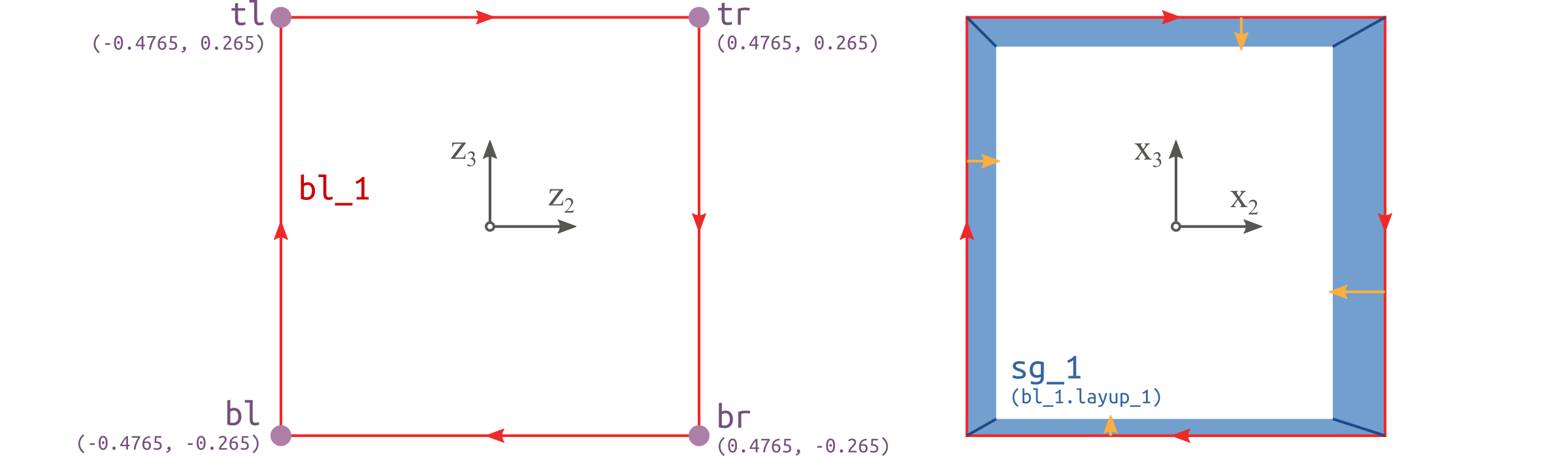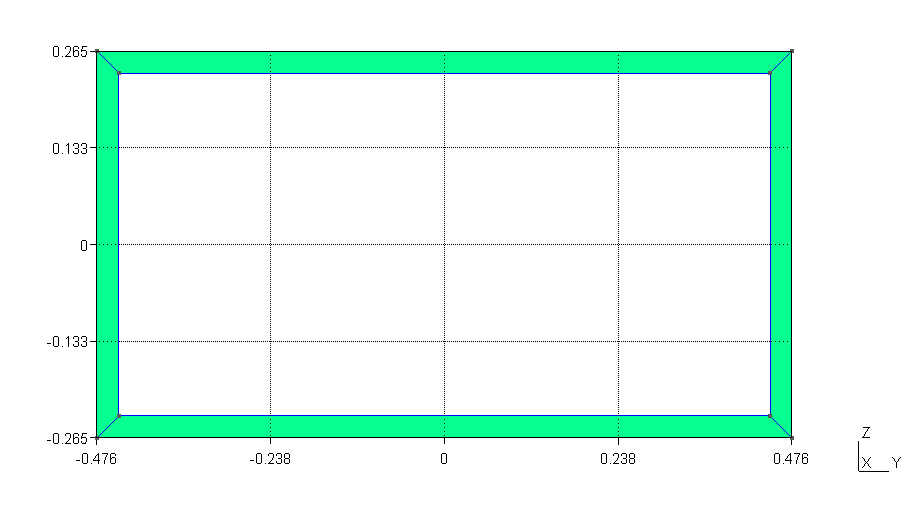# Box beam#

## Problem description#

This example is a thin-walled box beam whose cross section is depicted in Fig. 47 [YU2012]. The width $$a_2=0.953$$ in, height $$a_3=0.530$$ in, and thickness $$t=0.030$$ in. Each wall has six plies of the same composite material and the same fiber orientation of $$15^\circ$$. Material properties and layup scheme are listed in Table 41 and Table 42. Cross-sectional properties are given in Table 43 and compared with those in Ref. [YU2012]. The tiny differences are due to different meshes. Complete input files can be found in examples\ex_box\, including box.xml and materials.xml.Figure 48 Base points, Base lines and Segments of the box beam cross section.#Figure 49 Meshed cross section viewed in Gmsh.#
Table 41 Material properties#

Name

Density

$$E_{1}$$

$$E_{2}$$

$$E_{3}$$

$$G_{12}$$

$$G_{13}$$

$$G_{23}$$

$$\nu_{12}$$

$$\nu_{13}$$

$$\nu_{23}$$

$$\mathrm{lb\cdot sec^2/in^4}$$

$$10^6\ \mathrm{psi}$$

$$10^6\ \mathrm{psi}$$

$$10^6\ \mathrm{psi}$$

$$10^6\ \mathrm{psi}$$

$$10^6\ \mathrm{psi}$$

$$10^6\ \mathrm{psi}$$

mat_1

0.0001353

20.59

1.42

1.42

0.87

0.87

0.696

0.30

0.30

0.34

Table 42 Layups#

Name

Layer

Material

Ply thickness

Orientation

Number of plies

$$\mathrm{in}$$

$$\circ$$

layup1

1

mat_1

0.05

-15

6

## Result#

Table 43 Results#

Component

Value

Reference [YU2012]

$$S_{11}$$ [$$\mathrm{lb}$$]

$$\phantom{-}1.437 \times 10^6$$

$$\phantom{-}1.437 \times 10^6$$

$$S_{22}$$ [$$\mathrm{lb}$$]

$$\phantom{-}9.026 \times 10^4$$

$$\phantom{-}9.027 \times 10^4$$

$$S_{33}$$ [$$\mathrm{lb}$$]

$$\phantom{-}3.941 \times 10^4$$

$$\phantom{-}3.943 \times 10^4$$

$$S_{14}$$ [$$\mathrm{lb \cdot in}$$]

$$\phantom{-}1.074 \times 10^5$$

$$\phantom{-}1.074 \times 10^5$$

$$S_{25}$$ [$$\mathrm{lb \cdot in}$$]

$$-5.201 \times 10^4$$

$$-5.201 \times 10^4$$

$$S_{36}$$ [$$\mathrm{lb \cdot in}$$]

$$-5.635 \times 10^4$$

$$-5.635 \times 10^4$$

$$S_{44}$$ [$$\mathrm{lb \cdot in^2}$$]

$$\phantom{-}1.679 \times 10^4$$

$$\phantom{-}1.679 \times 10^4$$

$$S_{55}$$ [$$\mathrm{lb \cdot in^2}$$]

$$\phantom{-}6.621 \times 10^4$$

$$\phantom{-}6.621 \times 10^4$$

$$S_{66}$$ [$$\mathrm{lb \cdot in^2}$$]

$$\phantom{-}1.725 \times 10^5$$

$$\phantom{-}1.725 \times 10^5$$